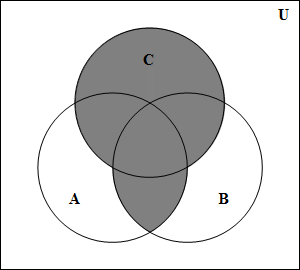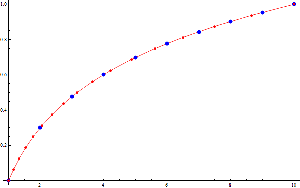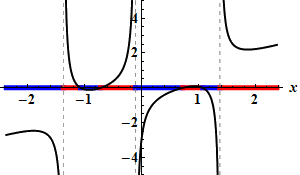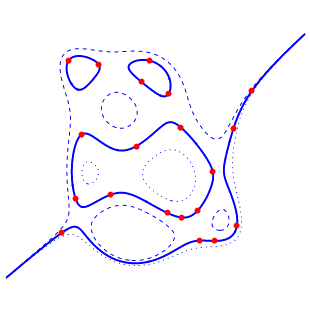### Mathematica Notebooks

Checked with1.Drawing Venn diagrams.

The notebook draws Venn diagrams for two or three sets. PDF version.

November 2011

2.Building and Using a Logarithmic Table.

How to compute square roots, build a logarithmic table, use a logarithmic table, improve accuracy by linear interpolation, and use slide rules. PDF version.

October 2011

3. Rearrangements of the Alternating Harmonic Series.

The notebook computes and visualizes rearrangements converging to a given limit. PDF version.

October 2011

4.Plotting Rational Functions.

October 2011

5. Algebraic Numbers.

An illustration of the use of the resultant to show that the set of algebraic numbers forms a field. PDF version.

April 2011

6.Conics, Cubics, etc.

The notebook plots the conic, cubic etc. passing through given points in the plane. PDF version.

March 2011

7. Decimal Representations.

The notebook computes the decimal representation of a given rational number. Representations in other bases can also be found. PDF version.

February 2011

8. The Cantor Function.